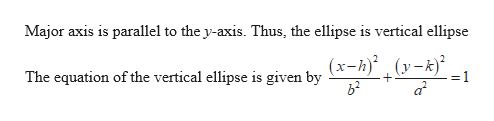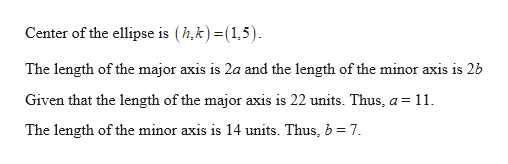# For Exercise, write the standard form of an equation of an ellipse subject to the given conditions. Major axis parallel to the y-axis; Center: (1, 5);Length of major axis: 22 units;Length of minor axis: 14 units.

Question
4 views

For Exercise, write the standard form of an equation of an ellipse subject to the given conditions.

Major axis parallel to the y-axis; Center: (1, 5);

Length of major axis: 22 units;

Length of minor axis: 14 units.

check_circle

Step 1help_outlineImage TranscriptioncloseMajor axis is parallel to the y-axis. Thus, the ellipse is vertical ellipse (x-h)' , (y-k)² The equation of the vertical ellipse is given by fullscreen
Step 2help_outlineImage TranscriptioncloseCenter of the ellipse is (h,k) =(1,5). The length of the major axis is 2a and the length of the minor axis is 2b Given that the length of the major axis is 22 units. Thus, a = 11. The length of the minor axis is 14 units. Thus, b = 7. fullscreen

### Want to see the full answer?

See Solution

#### Want to see this answer and more?

Solutions are written by subject experts who are available 24/7. Questions are typically answered within 1 hour.*

See Solution
*Response times may vary by subject and question.
Tagged in

### Other